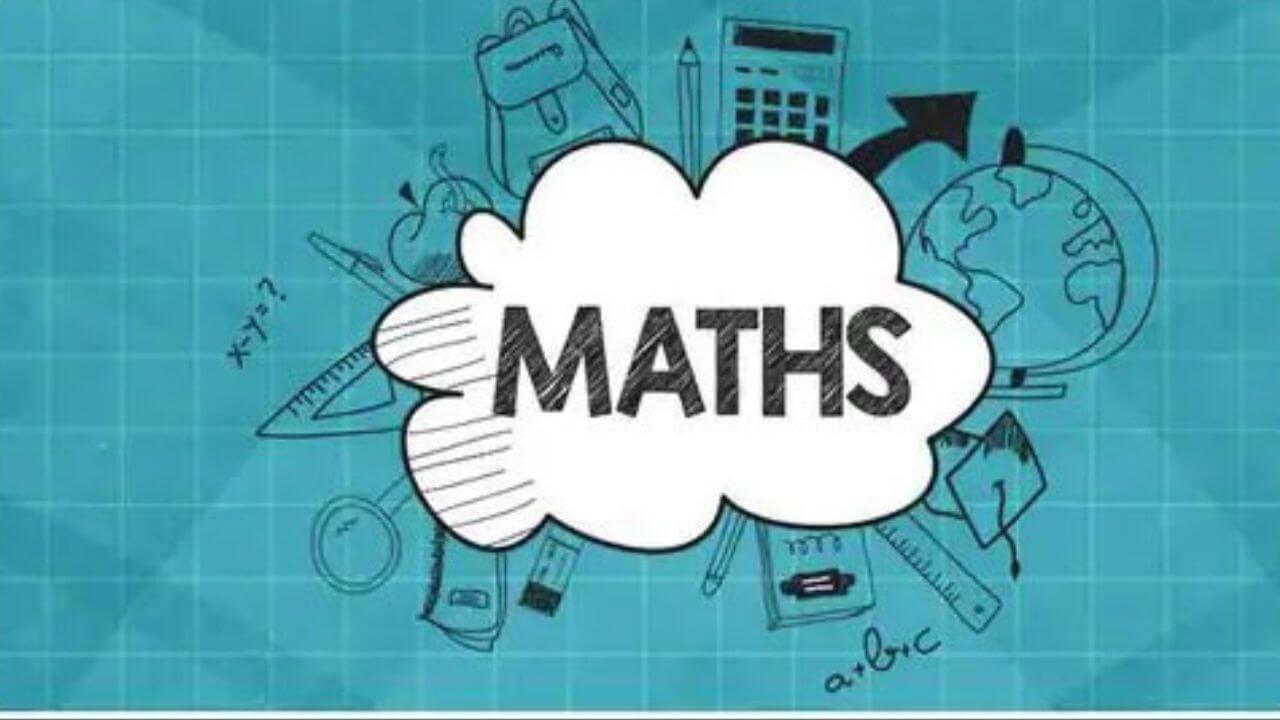# What Are the Basic Tips to be Clear About the Additive Inverse Concept?

The additive inverse of any kind of number is considered to be the value which on adding with original number will result in zero value. This is considered to be the Value that the people will be adding to a particular number to come out to the result as zero. Suppose a particular number is an original number then its additive inverse will be the minus of that particular number. Suppose additive inverse of 10 will be -10, the additive inverse of 9 will be -9, the additive inverse of 12 will be -12, and so on.

Additive inverse is also known as the opposite of the number, negation of the number, or change the sign of the original number in the whole process. It is very much important for the kids to be clear that the additive inverse of zero will only be the zero all the time without any kind of problem. This is considered to be the most important fact which the kids need to be clear about so that there is no hassle at any point in time and overall goals are very easily achieved.

## The additive inverse of any kind of number can be very easily found and the following are the basic steps to be taken into consideration by the people in this particular area:

1. Additive inverse of any given number can be found by changing the sign of it
2. The additive inverse of the positive number will be negative whereas the additive inverse of the negative number will be positive.
3. There will be no change in the numerical value in the whole process
4. People simply need to change the sign of the number without any kind of issue in the whole system
5. The additive inverse of 8 will be -8
6. The additive inverse of -6 will be 6
7. The addition of the number and its additive inverse will always be equal to the identity.

## Following are the basic properties associated with the concept of the additive inverse:

1. This particular property will simply make sure that changing the sign of the number will be carried out and people need to add it to the original number to get the answer is equal to 0
2. The properties of the additive inverse will be based upon the implementation of the concept that it will be the negation of the original number
3. Minus minus X will always become out to be X in this particular area
4. Minus X Square will always be coming out to X Square
5. Minus (X plus Y) = (-x) + (-y)
6. Several other kinds of related properties are also there in this particular area which the kids need to be clear about.

Whenever the additive inverse will be added to the original number the resultant will always be zero and this particular system can always be based upon rational numbers, integers, rational numbers, complex numbers, and irrational numbers without any kind of problem. It is also very much important for people to be clear about the concept of finding out the additive inverse of the natural or whole numbers so that there is no problem at any point in time.

Complex numbers are considered to be the combination of real numbers as well as imaginary numbers which is the main reason that people need to be clear about finding out the additive inverse of this particular system as well so that there is no problem at any point in time and people also need to be clear about the concept of finding the additive inverse of the rational numbers as well.

It is very much important for the kids to register themselves on platforms like Cuemath so that there is no problem at any point in time and overall goals are very easily achieved. Apart from this people need to be clear about the basic differences between additive inverse and multiplicative inverse so that there is no problem at any point in time and overall goals are very efficiently achieved without any kind of problem.